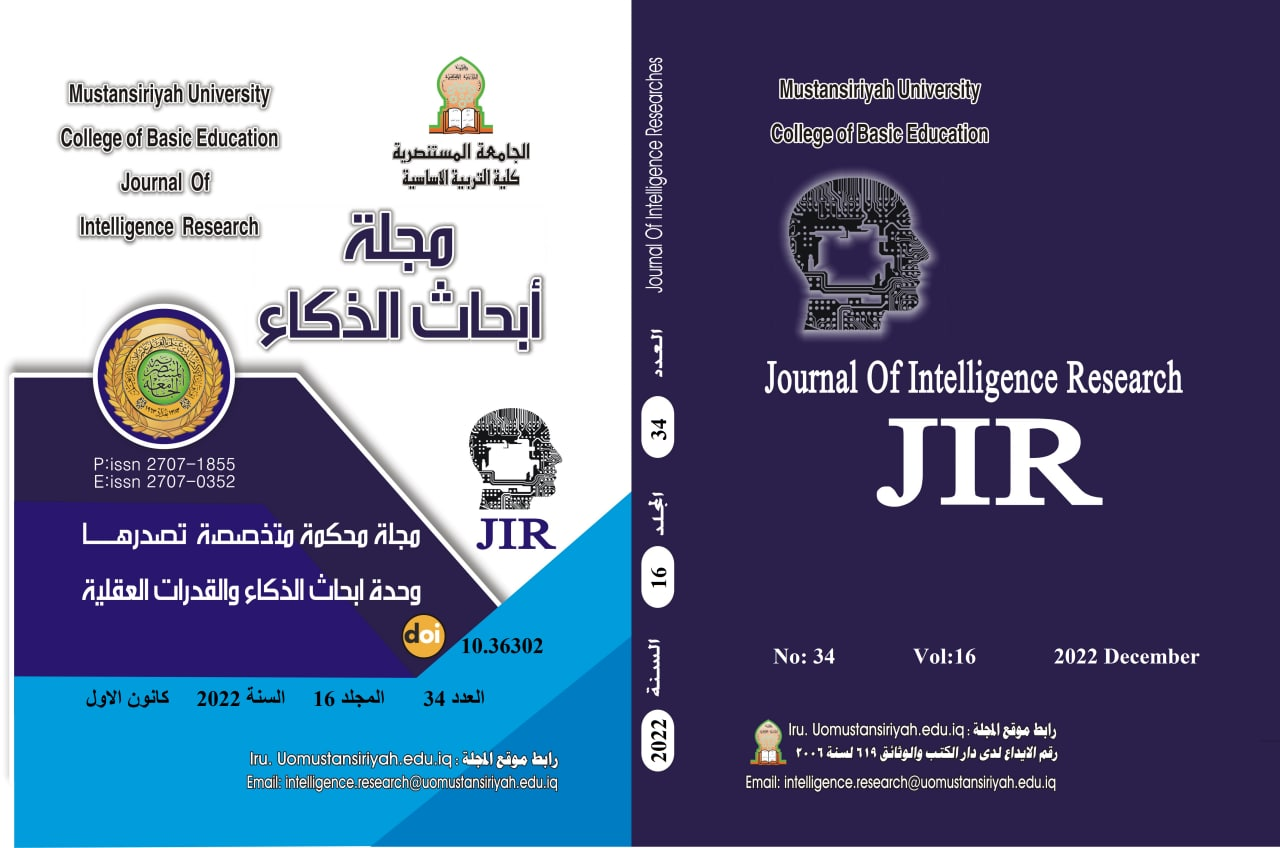# Mathematics flow and its relationship to the acquisition of mathematical concepts for male and female students of the sixth grade of primary school

## Authors

• ســـرى يونــس مجيــد
• أ. د. غالب خزعل محمد

## Keywords:

Keywords: Mathematical flow, acquiring mathematical concepts.

## Abstract

Abstract:

‏The current research aims to identify the mathematical flow and its relationship to the acquisition of mathematical concepts for male and female students of the sixth grade. The descriptive correlative method was adopted for the research. The research sample consisted of (600) male and female students from the sixth grade of primary school of the General Directorates of Education in Baghdad / Al-Rusafa Governorate (the first, the second, and the third) and Karkh (the first, the second, and the third). They were chosen at random for the second semester of the year (2021-2022) A.D. And then preparing the two search tools, which are: Mathematical Flow Scale, consisting of (10) items, Using the alpha-Cronbach equation, the value of the scale's stability coefficient was (0.885). And the testing of the athlete of the sporting concepts on three levels of each concept, which is (the definition of the concept, the distinction of the concept, the comprehension of the concept), the component of (78) is a subject of developed (choice of multiple) by (26)  Using the alpha-Cronbach equation, the value of the reliability coefficient of the test was (0.863). The results showed that students (primary school students in general have the mathematical flow, and there is no statistically significant difference between the grades of male and female students in the mathematical flow scale, in addition to the low percentage of acquiring mathematical concepts for the students of the male and female students in the male and female students’ test, and there is no difference in the academic achievement  Acquisition of mathematical concepts, and the presence of a weak direct correlation between the test of acquiring mathematical concepts and the measure of inclinations towards mathematics Several recommendations were reached, the most important of which is the acquisition of experiences for male and female students, each according to his abilities, and his mathematical flow leads to an increase in students' interest in mathematics.2023-01-12

## How to Cite

يونــس مجيــد س. ., & خزعل محمد أ. د. غ. . (2023). Mathematics flow and its relationship to the acquisition of mathematical concepts for male and female students of the sixth grade of primary school. مجلة أبحاث الذكاء, 16(34), 118–141. Retrieved from https://iru.uomustansiriyah.edu.iq/index.php/intel/article/view/423

المقالات